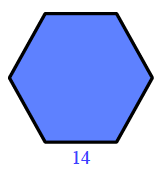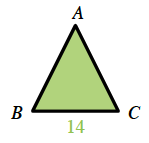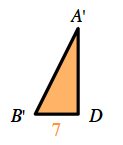### Home > INT2 > Chapter 11 > Lesson 11.1.1 > Problem11-7

11-7.

A regular hexagonal prism has a volume of $2546.13$ cm$3$ and the base has an edge length of $14$ cm. What are the height and surface area of the prism?

Start by finding the area of the base of the prism

Divide the hexagon into six triangles.

Find $∠A$ by dividing the central angle of the hexagon by $6$.

Divide the triangles in half to make two right triangles.

Solve for unknown angles. Is this a special triangle?

When you find the area of the $30$-$60$-$90$ triangle, multiply it by $12$ in order to find the area of the hexagon.

$\text{Volume} = (\text{area of the base})(\text{height})$
$2546.12 \text{ cm}^3 = (509.23 \text{ cm}^2)(\text{height})$

$\frac{360º}{6\text{ triangles}}=60º$

$m∠A = 60º$

$\angle A'=\frac{60º}{2}$

$m∠A' = 30º$

$\angle B'=180º-30º-90º$

$m∠B' = 60º$Once you have the height of the prism, you have all the information necessary to find the surface area.